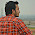Electrical Engineering Basics Questions

Today we are Going to Provide You Electrical engineering interview questions from Electrical Engineering Basics Chapter.

All matter is composed of - Atoms

To be a conductor, the substance must contain some mobile electrons

Conductivity depends on the number of electrons. - (Yes/No)

The energy level of an electron decreases as its distance from the nucleus increases.  - (Yes/No)

Atoms with fewer than four valence electrons are good conductors.

Atoms with more than four valence electrons are poor conductors.

Atoms with four valence electrons are - semi-conductors

Mass of an electron is approximately equal to 1/1000 mass of the proton - (Yes/No)

The diameter of an electron = 10-15 Meter (Yes/No)

When an electron is removed from a neutral atom, this atom becomes positively charged and is called a - Positive Ion

The controlled movement of electrons through a substance is called - Current

Electromotive force is the force that causes a current of electricity to flow.

Also Read - Basic of Electrical Engineering and Basic Electrical Formulas

The is a unit of potential difference and electromotive force - Volt

Electron-volt is a unit in terms of which the energies of atomic particles are expressed. (Yes/No)

The opposition to the flow of electrons is called electrical resistance

The practical unit of electric resistance is mho. (Yes/No)

Resistivity of a material may be defined as the resistance between the opposite faces of a meter cube of that material.

The reciprocal of specific resistance of a material is called its conductivity

The unit of conductivity is ohm/ meter. (Yes/No)

The resistance of metal conductors increases with rising of temperature. (Yes/No)

The resistance of semiconductors and all electrolytes decreases as the temperature rises.

A linear resistor is one which Obey's Ohm's law.

At very low temperature, some metals acquire zero electrical resistance and zero magnetic induction, the property known as super-conductivity

A fixed resistor is the simplest type of resistor and its value is constant and unchangeable.

Also ReadImportant Topic on A.C. Fundamentals

A variable resistor is commonly called a control. (Yes/No)

The most common type of special resistor is the fusible type. (Yes/No)

In a series combination of resistors, the current flowing through each resistor is different. (Yes/No

The resistivity of pure semiconductors is of the order of 1 ohm-meters. (Yes/No)

The symbol marked R8 represents a fixed resistor.

The part represented by the symbol marked R1 is a Variable resistor.

This is Part 1 of Electrical Engineering Basics Questions with Answers, these Questions and Answers are Very Important For Your Exam and Interview. Keep Visiting ElectricalNotes4u for Latest Electrical Engineering MCQ, Notes, Interview Questions with Answers.

Share:

1.1.